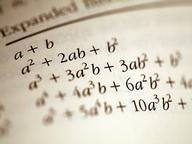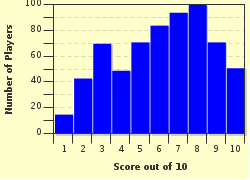FREE! Click here to Join FunTrivia. Thousands of games, quizzes, and lots more!# Algebra Wonderland! Trivia Quiz

### Calculators are allowed, but you will find that it will not be very useful on most problems. Have fun! Note: be on the look out for hidden words in questions!

A multiple-choice quiz by methane. Estimated time: 6 mins.

Author
methane
Time
6 mins
Type
Multiple Choice
Quiz #
284,632
Updated
Dec 03 21
# Qns
10
Difficulty
Tough
Avg Score
6 / 10
Plays
2573
1. Let's start out with food! There is one chocolate mint bar with plenty of sprinkles on it. Obe Seguy and his 4 friends want to buy it. They buy it using their combined money that totaled to \$2.50. If they decide to split it into equal parts for each one of them, what is the percentage of the whole chocolate bar each of the kids will receive?

#### NEXT>

2. What is the y-intercept of a direct variation? A direct variation always passes through the origin.

Answer: (The hint is the second sentence in the question.)

#### NEXT>

3. The sum of two numbers is 74. The difference between the two numbers is 16. What are the two numbers?

Answer: (Two numbers with "and" between them.)

#### NEXT>

4. Don T. Benice has a pattern of cans. The first can has 2 balls inside. The second has 5 markers. The third has 8 bananas, and the fourth has 11 children captured inside. When "n" represents the position of the can in his collection (the can with markers would be 2 since it is the second can), which of the following shows the amount of objects in each can? Hint

3n+(12-8)
3
3n
3n-1

#### NEXT>

5. Skinny Knee is drawing a graph representing the growth of his knees over the past year. He found that following equation, 4x+2y=6, could be used to represent the line in his graph. Using this information, which equation is perpendicular to his line? Hint

y=-2x+6
3x-6y=5
6y+2x=1
None of the choices are correct.

#### NEXT>

6. Notve Rysmart is in debt of 6 dollars. In one year, his debt multiplies by the same amount. In the year after that, it again multiplies by the same amount he had to begin with. How much debt is Notve in now? Hint

He has \$216
\$216
\$219
He has paid off his debt, and now has \$0.

#### NEXT>

7. "k" represents the slope in a direct variation equation.

True
False

#### NEXT>

8. Mr. Po jumps for joy when he gets TWO bottles of soda in a broken vending machine when pressed in one key, D1. Does this represent a function?

Yes
No

#### NEXT>

9. Skinny Knee drew another graph. This time, it shows how the width of his knees had narrowed over a year. He figured out that y=2x+12 represents the line in his graph. Based on that, which of the following equations is parallel to his line? Hint

y=12x+2
x=x+6
It doesn't matter!
y=2x+24

#### NEXT>

10. Which of the following is equal to: 2^3*2^10? Thank you for taking my quiz and please leave a rating! Hint

2^13
4^13
20
Both 20 and 2^13

 (Optional) Create a Free FunTrivia ID to save the points you are about to earn:Select a User ID:Choose a Password:Your Email:

Most Recent Scores
Sep 26 2023 : cseanymph: 7/10
Sep 23 2023 : AdamM7: 7/10

Score DistributionQuiz Answer Key and Fun Facts
1. Let's start out with food! There is one chocolate mint bar with plenty of sprinkles on it. Obe Seguy and his 4 friends want to buy it. They buy it using their combined money that totaled to \$2.50. If they decide to split it into equal parts for each one of them, what is the percentage of the whole chocolate bar each of the kids will receive?

There are five people (Obe with his 4 friends). Divide one (the whole chocolate bar) by five, and .2 is the answer. After converting this into a percent, the answer is 20%.
2. What is the y-intercept of a direct variation? A direct variation always passes through the origin.

A direct variation is a line that always passes through the origin (0,0)! The y-intercept is the point which the line crosses the y-axis.
3. The sum of two numbers is 74. The difference between the two numbers is 16. What are the two numbers?

Solve by using system of equations. The two equations are x+y=74 and x-y=16. Isolate either y or x in the second equation and substitute in the first.
4. Don T. Benice has a pattern of cans. The first can has 2 balls inside. The second has 5 markers. The third has 8 bananas, and the fourth has 11 children captured inside. When "n" represents the position of the can in his collection (the can with markers would be 2 since it is the second can), which of the following shows the amount of objects in each can?

The coefficient is 3, and the constant is 1. Setting up a chart is a time-saving way of solving this problem. If you multiply 3 by the position of the can and subtract 1 you will get the amount of objects inside. Let's say it is the can with markers, so 3(2)-1=5. 5 markers are inside!
5. Skinny Knee is drawing a graph representing the growth of his knees over the past year. He found that following equation, 4x+2y=6, could be used to represent the line in his graph. Using this information, which equation is perpendicular to his line?

First convert the equation in the problem, 4x+2y=6, into y-intercept form. y=-2x+3 is the result. Perpendicular equations will have the opposite reciprocal of the slope. 2's opposite reciprocal is -1/2, so 3x-6y=5 is correct.
6. Notve Rysmart is in debt of 6 dollars. In one year, his debt multiplies by the same amount. In the year after that, it again multiplies by the same amount he had to begin with. How much debt is Notve in now?

\$6 times \$6 times \$6 equals -\$216. This could be represented by \$6^3.
7. "k" represents the slope in a direct variation equation.

It is not certain yet why it is, but it just is. "m" represents the slope in other scenarios, but here, it is "k".
8. Mr. Po jumps for joy when he gets TWO bottles of soda in a broken vending machine when pressed in one key, D1. Does this represent a function?

A function occurs when there is only ONE y value, in this case is the soda bottles, for each x vale, in this case is the key D1. In this scenario, Mr. Po received two bottles, which stops this from being a function.
9. Skinny Knee drew another graph. This time, it shows how the width of his knees had narrowed over a year. He figured out that y=2x+12 represents the line in his graph. Based on that, which of the following equations is parallel to his line?

To find the parallel equation, look at the slope. Parallel lines have the same slope, which in this case is 2. "It doesn't matter" is not correct, because this is a factor on your score of this quiz.
10. Which of the following is equal to: 2^3*2^10? Thank you for taking my quiz and please leave a rating!

To combine exponents with the same base, just add the exponents and keep the base the same. 3+10=13... so 2^13 is the answer.
Source: Author methane

This quiz was reviewed by FunTrivia editor crisw before going online.
Any errors found in FunTrivia content are routinely corrected through our feedback system.
Related Quizzes
1. Linear Equations in One Variable Average